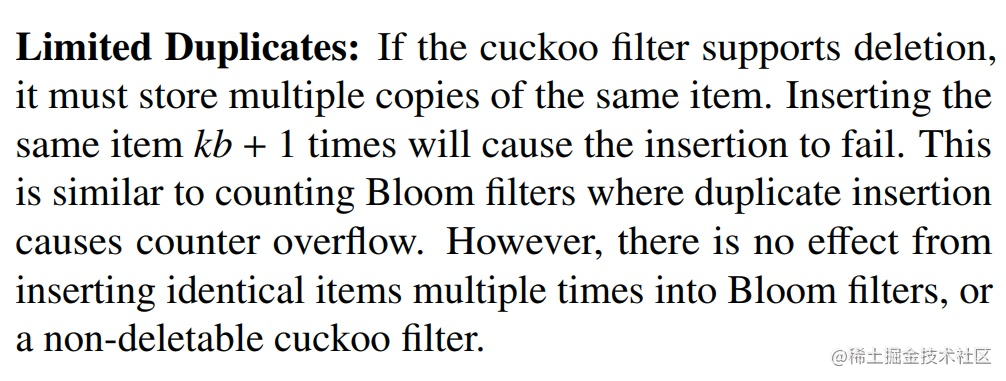# 布隆过滤器过时了，未来属于布谷鸟过滤器？``````p1 = hash1(x) % l
p2 = hash2(x) % l

``````fp = fingerprint(x)
p1 = hash1(x) % l
p2 = hash2(x) % l

``````fp = fingerprint(x)
p1 = hash(x)
p2 = p1 ^ hash(fp)  // 异或

``````p1 = p2 ^ hash(fp)

``````// l = power(2, 8)
p_ = p & 0xff

``````type bucket byte  // 一个桶，4个座位
type cuckoo_filter struct {
buckets [size]bucket // 一维数组
nums int  // 容纳的元素的个数
kick_max  // 最大挤兑次数
}

``````def insert(x):
fp = fingerprint(x)
p1 = hash(x)
p2 = p1 ^ hash(fp)
// 尝试加入第一个位置
if !buckets[p1].full():
nums++
return true
// 尝试加入第二个位置
if !buckets[p2].full():
nums++
return true
// 随机挤兑一个位置
p = rand(p1, p2)
c = 0
while c < kick_max:
// 挤兑
old_fp = buckets[p].replace_with(fp)
fp = old_fp
// 计算对偶位置
p = p ^ hash(fp)
// 尝试加入对偶位置
if !buckets[p].full():
nums++
return true
c++
return false

``````def contains(x):
fp = fingerprint(x)
p1 = hash(x)
p2 = p1 ^ hash(fp)
return buckets[p1].contains(fp) || buckets[p2].contains(fp)

``````def delete(x):
fp = fingerprint(x)
p1 = hash(x)
p2 = p1 ^ hash(fp)
ok = buckets[p1].delete(fp) || buckets[p2].delete(fp)
if ok:
nums--
return ok

so far so good！布谷鸟过滤器看起来很完美啊！删除功能和获取元素个数的功能都具备，比布隆过滤器强大多了，而且似乎逻辑也非常简单，上面寥寥数行代码就完事了。如果插入操作返回了 false，那就意味着需要扩容了，这也非常显而易见。

but! 考虑一下，如果布谷鸟过滤器对同一个元素进行多次连续的插入会怎样？这句话明确告诉我们如果想要让布谷鸟过滤器支持删除操作，那么就必须不能允许插入操作多次插入同一个元素，确保每一个元素不会被插入多次（kb+1）。这里的 k 是指 hash 函数的个数 2，b 是指单个位置上的座位数，这里我们是 4。

``````go get github.com/seiflotfy/cuckoofilter

``````package main

import (
"fmt"
"github.com/seiflotfy/cuckoofilter"
)

func main() {
cf := cuckoo.NewFilter(100000)
for i := 0; i < 15; i++ {
var ok = cf.Insert([]byte("geeky ogre"))
fmt.Println(ok)
}
}

-------
true
true
true
true
true
true
true
true
false
false
false
false
false
false
false

Redis 布谷鸟过滤器模块： github.com/kristoff-it…